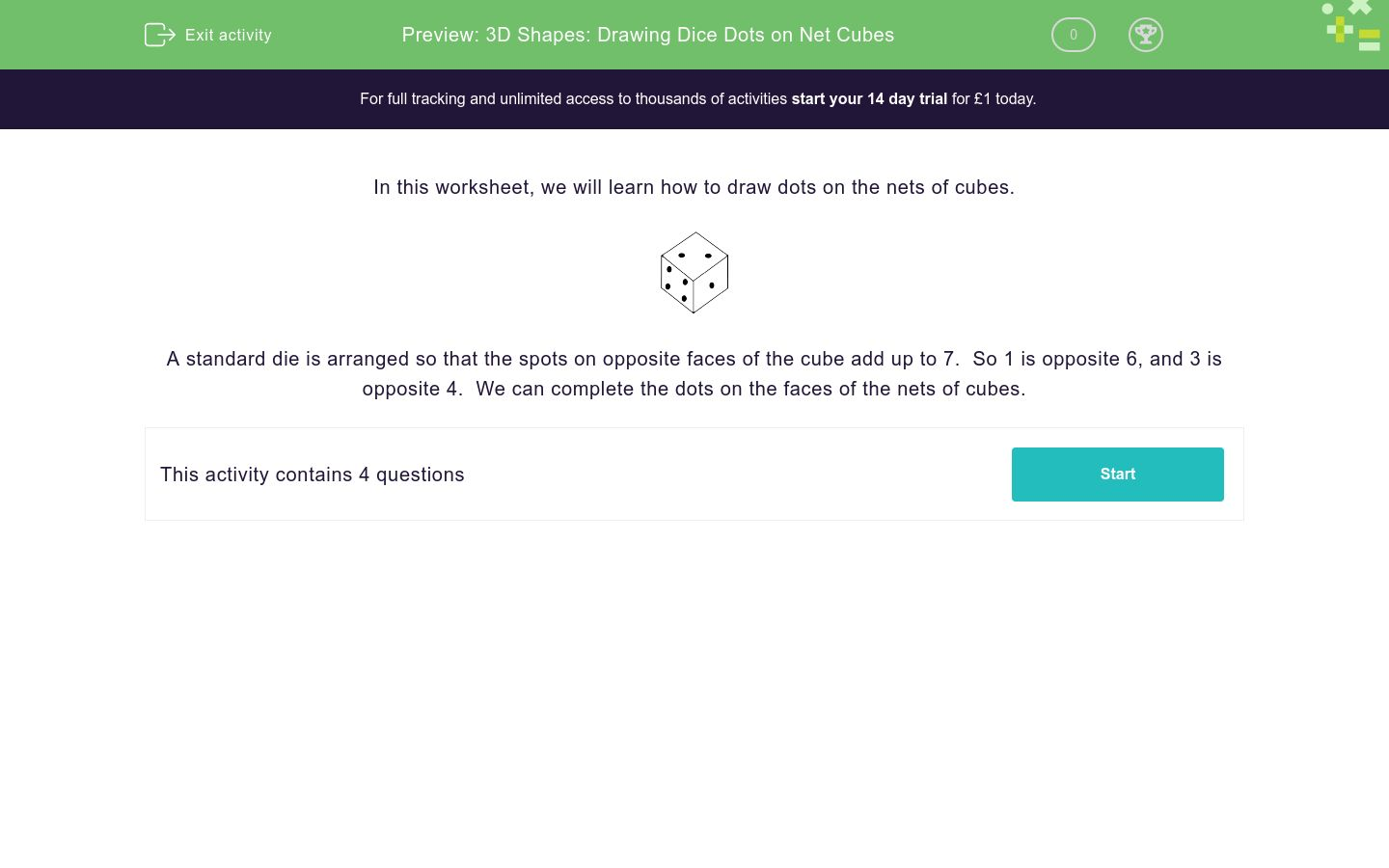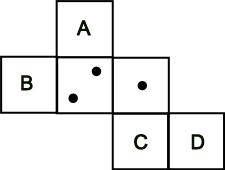# 3D Shapes: Drawing Dice Dots on Net Cubes

In this worksheet, students are directed to place dice dots and nets of cubes.Key stage:  KS 2

Curriculum topic:   Geometry: Properties of Shapes

Curriculum subtopic:   Know 3D Shapes

Difficulty level:### QUESTION 1 of 10

In this worksheet, we will learn how to draw dots on the nets of cubes.A standard die is arranged so that the spots on opposite faces of the cube add up to 7.  So 1 is opposite 6, and 3 is opposite 4.  We can complete the dots on the faces of the nets of cubes.

If A = 1 and B = 2, work out what C and D must be.## Column B

C
6
D
5

If A = 3, work out what B, C and D must be.## Column B

B
5
C
6
D
4

If A =3 and B = 1, work out what C and D must be.## Column B

C
6
D
4

If A = 1 and B = 3, work out what C and D must be.## Column B

C
4
D
6
• Question 1

If A = 1 and B = 2, work out what C and D must be.## Column B

C
6
D
5
• Question 2

If A = 3, work out what B, C and D must be.## Column B

B
6
C
4
D
5
• Question 3

If A =3 and B = 1, work out what C and D must be.## Column B

C
4
D
6
• Question 4

If A = 1 and B = 3, work out what C and D must be.## Column B

C
6
D
4
---- OR ----

Sign up for a £1 trial so you can track and measure your child's progress on this activity.

### What is EdPlace?

We're your National Curriculum aligned online education content provider helping each child succeed in English, maths and science from year 1 to GCSE. With an EdPlace account you’ll be able to track and measure progress, helping each child achieve their best. We build confidence and attainment by personalising each child’s learning at a level that suits them.

Get started